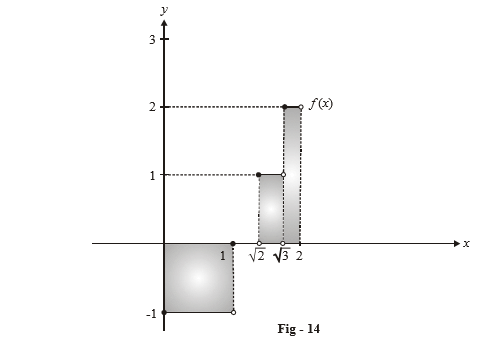# Basic Examples on Definite Integrals Set 5

Go back to  'Definite Integration'

Example –10

Prove that \begin{align}\int\limits_0^\pi {\frac{{\sin \left( {2n + 1} \right)x}}{{\sin x}}} \,dx = \pi \,\,{\text{for all }}n \in \mathbb{N}\end{align}

Solution: Let $${I_n} = \int\limits_0^\pi {\frac{{\sin \left( {2n + 1} \right)x}}{{\sin x}}} \,dx.$$

What is I1 ?

\begin{align}&{I_1} = \int\limits_0^\pi {\frac{{\sin 3x}}{{\sin x}}} \,dx\\\,\,\,\,\,&\quad= \int\limits_0^\pi {\left( {3 - 4{{\sin }^2}x} \right)} \,dx\\\,\,\,\, &\quad= \int\limits_0^\pi {\left( {3 - 2\left( {1 - \cos 2x} \right)} \right)} \,dx\\\,\,\,\,\,&\quad\left. { = \left( {x + \sin 2x} \right)} \right|_0^\pi \\\,\,\,\,\, &\quad= \pi\end{align}

Thus, n = 1 satisfies the stated property. How do we approach the general case?

Some reflection on the nature of the integral will show you that evaluating  $$I_n$$   by itself would be tedius. What we could instead do is this:

We have already shown that $${I_1} = \pi .$$ If we show that $${I_{n + 1}} - {I_n} = 0,$$ our task would be accomplished, since then $${I_2},\,\,{I_3}$$ and all the higher order integrals become equal to I 1 , which is $$\pi \,;$$ this is what we want to prove.

\begin{align}&{I_{n + 1}} - {I_n} = \int\limits_0^\pi {\left\{ {\frac{{\sin \left( {2n + 3} \right)x}}{{\sin x}} - \frac{{\sin \left( {2n + 1} \right)x}}{{\sin x}}} \right\}} \,dx\\\,\,\,\,\,\,\,\,\,\,\,\,\,\,\,\,\,\, &\qquad\qquad= 2\int\limits_0^\pi {\frac{{\,\,\,{\mathop{\rm si}\nolimits} x\cos \left( {2n + 2} \right)x}}{{{\mathop{\rm si}\nolimits} x}}} \,dx\\\,\,\,\,\,\,\,\,\,\,\,\,\,\,\,\,\,\, &\qquad\qquad= \frac{1}{{n + 1}}\left. {\left\{ {\sin \left( {2n + 2} \right)x} \right\}} \right|_0^\pi \\\,\,\,\,\,\,\,\,\,\,\,\,\,\,\,\,\,\, &\qquad\qquad= 0\end{align}

Therefore,

${I_n} = \pi \,\,\,\,\forall \,\,n \in \,\,N$

Example –11

Evaluate $$\int\limits_0^{\pi /2} {\ln \left( {\tan x} \right)dx}$$

Solution: Careful observation will show that the function $$\ln \left( {\tan x} \right)$$ is odd about $$x = \frac{\pi }{4},$$ because,

\begin{align}\ln \left( {\tan \left( {\frac{\pi }{2} - x} \right)} \right) = \ln \left( {\cot x} \right) = \ln \left( {\frac{1}{{\tan x}}} \right) = - \ln \left( {\tan x} \right)\end{align}

Thus, as discussed in property –7, the given integral will become 0.

Example –12

Evaluate $$\int\limits_0^2 {\left[ {{x^2} - 1} \right]} \,dx.$$

Solution: The function to be integrated as been sketched below in the region of interest:This function is discontinuous; as discussed in property-3, we can split the required interval of integration. We will do it in such a way so that in each of the sub-intervals that we obtain, the function is continuous and can be integrated.

Thus, if $$\left[ {{x^2} - 1} \right] = f\left( x \right),\,\,{\rm{then}}$$

\begin{align}&\int\limits_0^2 {f\left( x \right)dx} = \int\limits_0^1 {f\left( x \right)dx + \int\limits_1^{\sqrt 2 } {f\left( x \right)dx + \int\limits_{\sqrt 2 }^{\sqrt 3 } {f\left( x \right)dx + } \int\limits_{\sqrt 3 }^2 {f\left( x \right)dx} } } \\\,\,\,\,\,\,\,\,\,\,\,\,\,\,\,\,\,\,\,\,\, &\qquad\;\;\;\quad\quad= \int\limits_0^1 {\left( { - 1} \right)dx + \int\limits_1^{\sqrt 2 } {\left( 0 \right)dx + } \int\limits_{\sqrt 2 }^{\sqrt 3 } {\left( 1 \right)dx + } \int\limits_{\sqrt 3 }^2 {\left( 2 \right)dx} } \\\,\,\,\,\,\,\,\,\,\,\,\,\,\,\,\,\,\,\,\,\, &\qquad\;\;\;\quad\quad= - 1 + 0 + \left( {\sqrt 3 - \sqrt 2 } \right) + 2 \cdot \left( {2 - \sqrt 3 } \right)\\\,\,\,\,\,\,\,\,\,\,\,\,\,\,\,\,\,\,\,\,\, &\qquad\;\;\;\quad\quad= 3 - \left( {\sqrt 2 + \sqrt 3 } \right)\end{align}

In general, for any discontinuous function f(x) whose integral we need to evaluate, the approach described above is followed.  f(x) is separately integrated in sub–intervals where it is continuous and the results so obtained are added.

## TRY YOURSELF - I

Q. 1   Prove that \begin{align}\int\limits_0^\pi {\frac{{\sin 2nx}}{{\sin x}}} \,dx = 0\,\,\,\,\forall \,\,n \in \mathbb{N}\end{align}

Q. 2   If n is an odd positive integer, prove that

${I_n} = \int\limits_0^{\pi /2} {\frac{{\sin nx}}{{\sin x}}} \,dx = \frac{\pi }{2}$

Q. 3   Show that\begin{align}\int\limits_0^{\pi /4} {{{\left( {\cos 2\theta } \right)}^{3/2}}\cos \theta } \,d\theta = \frac{{3\pi }}{{16\sqrt 2 }}\end{align}

Q. 4   Show that \begin{align}\int\limits_0^{\pi /3} {\frac{x}{{1 + \sec x}}\,\,dx = \,\frac{{{\pi ^2}}}{{18}}} - \frac{\pi }{{3\sqrt 3 }} + 2\ln \frac{2}{{\sqrt 3 }}\end{align}

Q. 5   For x > 0, if $$f\left( x \right) = \int\limits_1^x {\frac{{\ln z}}{{{z^2} + z + 1}}dz,}$$ show that $$f\left( x \right) = f\left( {\frac{1}{x}} \right)$$

Q. 6   If $${I_n} = \int\limits_0^\pi {\frac{{1 - \cos nx}}{{1 - \cos x}}} \,\,dx\,$$ where n is a non-negative integer, show that $${I_n},{I_{n + 1}}\,\,{\rm{and}}\,\,{I_{n + 2}}$$ are in A.P.

Q. 7   Show that $$\int\limits_a^b {\frac{1}{{\sqrt {\left( {x - a} \right)\left( {b - x} \right)} }}dx} ,\,\,b > a\,\,{\rm{is}}\,\,$$ equal to $$\pi$$ .

Q. 8   If I n = \begin{align}\int\limits_0^{\pi /2} {{{\cos }^n}x\,dx,n \in N,}\end{align} show that \begin{align}{I_n} = \frac{{n - 1}}{n}{I_{n - 2}}\end{align}

Q. 9   Prove that \begin{align}\int\limits_0^\pi {\frac{1}{{3 + 2\sin x + \cos x}}dx = \frac{\pi }{4}}\end{align}

Q. 10   Prove that \begin{align}\int\limits_2^3 {\frac{{2{x^5} + {x^4} - 2{x^3} + 2{x^2} + 1}}{{\left( {{x^2} + 1} \right)\left( {{x^4} - 1} \right)}} = \frac{{\ln 6}}{2} - \frac{1}{{10}}}\end{align}

* You must have used substitutions in some of the questions above. Think about the validity of these substitutions. Is a substitution always valid? Or do we need to fulfill certain requirements if a substitution is to be valid?